# Intro to Statistics AP BIOLOGY Statistics Statistical analysis

• Slides: 17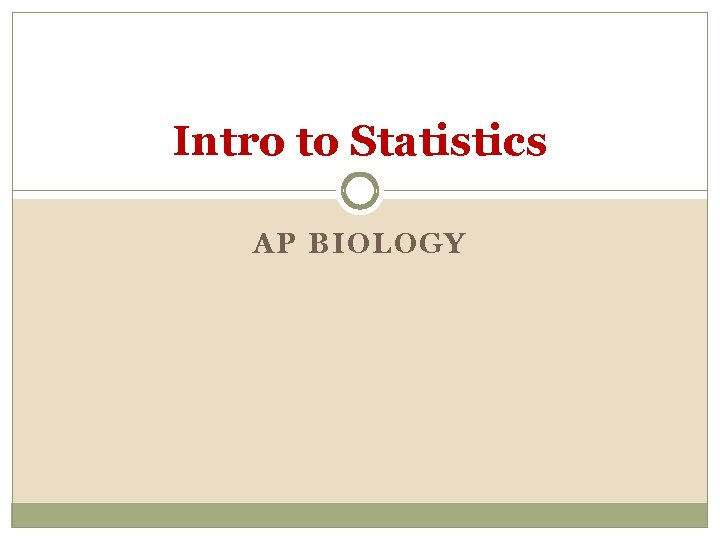Intro to Statistics AP BIOLOGY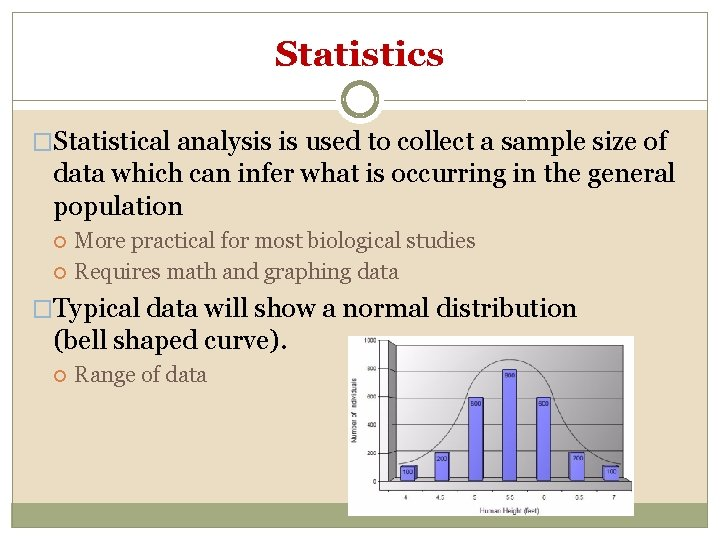Statistics �Statistical analysis is used to collect a sample size of data which can infer what is occurring in the general population More practical for most biological studies Requires math and graphing data �Typical data will show a normal distribution (bell shaped curve). Range of dataStatistical Analysis �Two important considerations How much variation do I expect in my data? What would be the appropriate sample size?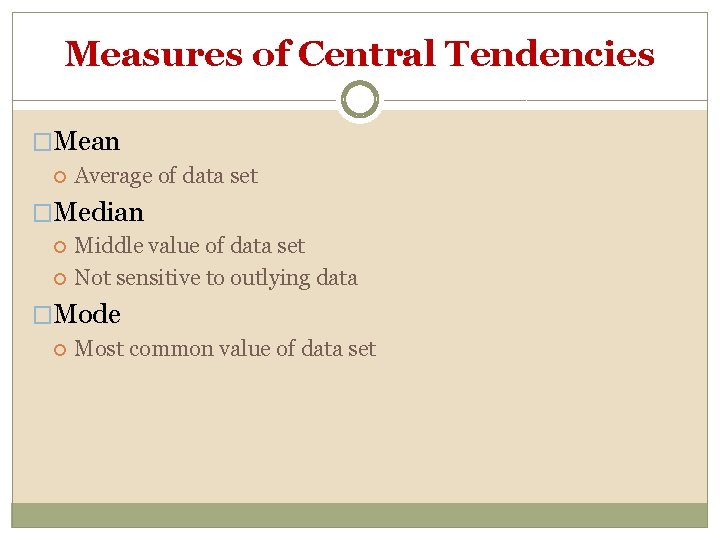Measures of Central Tendencies �Mean Average of data set �Median Middle value of data set Not sensitive to outlying data �Mode Most common value of data set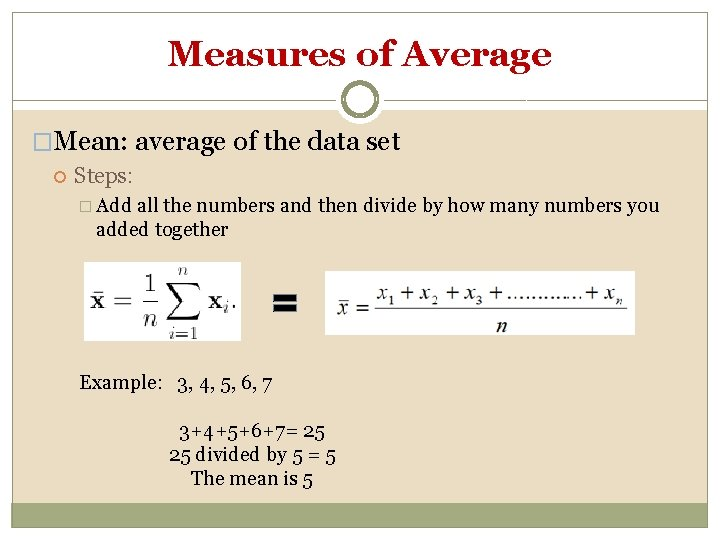Measures of Average �Mean: average of the data set Steps: � Add all the numbers and then divide by how many numbers you added together Example: 3, 4, 5, 6, 7 3+4+5+6+7= 25 25 divided by 5 = 5 The mean is 5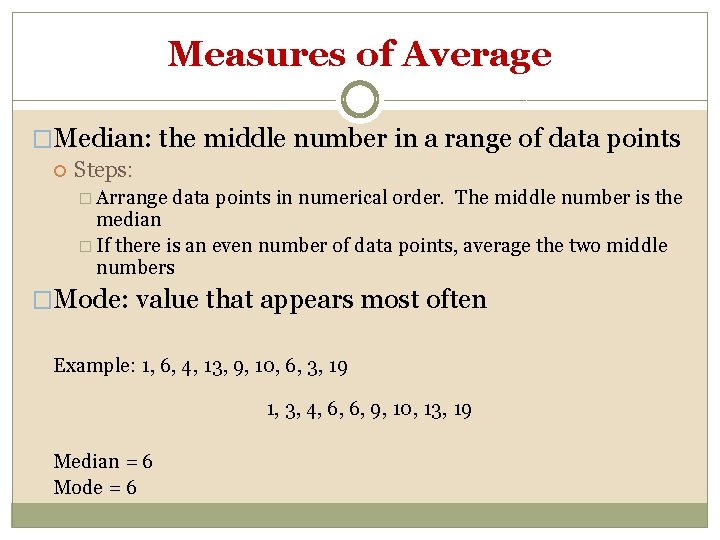Measures of Average �Median: the middle number in a range of data points Steps: � Arrange data points in numerical order. The middle number is the median � If there is an even number of data points, average the two middle numbers �Mode: value that appears most often Example: 1, 6, 4, 13, 9, 10, 6, 3, 19 1, 3, 4, 6, 6, 9, 10, 13, 19 Median = 6 Mode = 6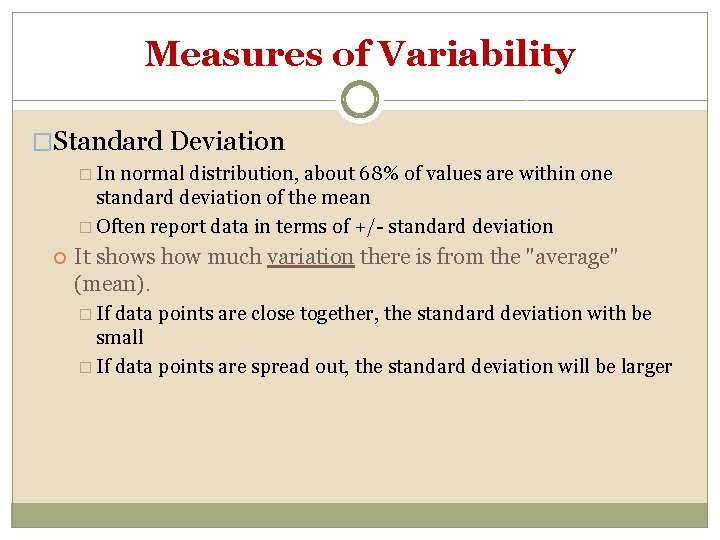Measures of Variability �Standard Deviation � In normal distribution, about 68% of values are within one standard deviation of the mean � Often report data in terms of +/- standard deviation It shows how much variation there is from the "average" (mean). � If data points are close together, the standard deviation with be small � If data points are spread out, the standard deviation will be larger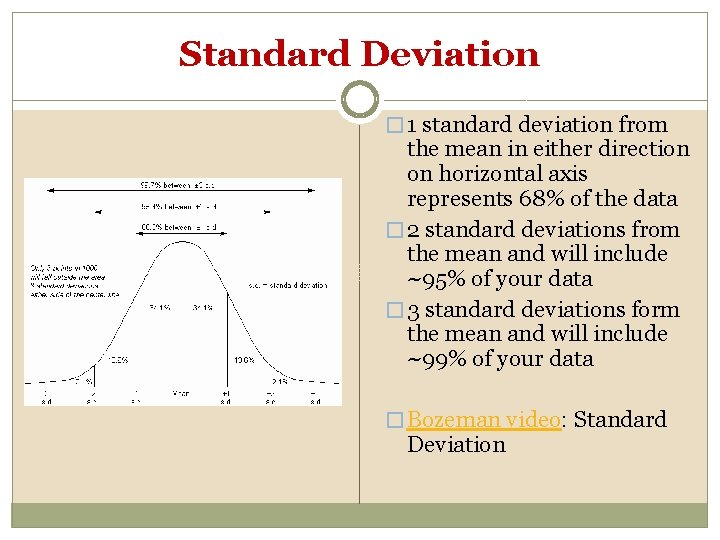Standard Deviation � 1 standard deviation from the mean in either direction on horizontal axis represents 68% of the data � 2 standard deviations from the mean and will include ~95% of your data � 3 standard deviations form the mean and will include ~99% of your data � Bozeman video: Standard DeviationCalculating Standard DeviationCalculating Standard Deviation Grades from recent quiz in AP Biology: 96, 93, 90, 88, 86, 84, 80, 70 1 st Step: find the mean (X) Measured Number Value x (x - X) 1 96 9 2 96 9 3 92 5 4 90 3 5 88 1 6 86 -1 7 86 -1 8 84 -3 9 80 -7 10 70 -17 TOTAL 868 TOTAL Mean, X 87 Std Dev (x - X)2 81 81 25 9 1 1 1 9 49 289 546Calculating Standard Deviation 2 nd Step: determine the deviation from the mean for each grade then square it Measured Number Value x (x - X) 1 96 9 2 96 9 3 92 5 4 90 3 5 88 1 6 86 -1 7 86 -1 8 84 -3 9 80 -7 10 70 -17 TOTAL 868 TOTAL Mean, X 87 Std Dev (x - X)2 81 81 25 9 1 1 1 9 49 289 546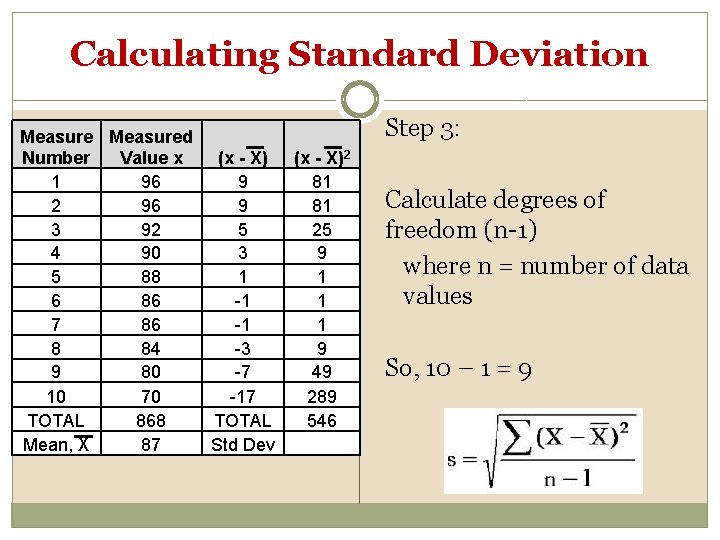Calculating Standard Deviation Measured Number Value x (x - X) 1 96 9 2 96 9 3 92 5 4 90 3 5 88 1 6 86 -1 7 86 -1 8 84 -3 9 80 -7 10 70 -17 TOTAL 868 TOTAL Mean, X 87 Std Dev Step 3: (x - X)2 81 81 25 9 1 1 1 9 49 289 546 Calculate degrees of freedom (n-1) where n = number of data values So, 10 – 1 = 9Calculating Standard Deviation Measured Number Value x (x - X) 1 96 9 2 96 9 3 92 5 4 90 3 5 88 1 6 86 -1 7 86 -1 8 84 -3 9 80 -7 10 70 -17 TOTAL 868 TOTAL Mean, X 87 Std Dev Step 4: (x - X)2 81 81 25 9 1 1 1 9 49 289 546 8 Put it all together to calculate S S = √(546/9) = 7. 79 =8Calculating Standard Error �So for the class data: Mean = 87 Standard deviation (S) = 8 � 1 s. d. would be (87 – 8) thru (87 + 8) or 81 -95 So, 68. 3% of the data should fall between 81 and 95 � 2 s. d. would be (87 – 16) thru (87 + 16) or 71 -103 So, 95. 4% of the data should fall between 71 and 103 � 3 s. d. would be (87 – 24) thru (87 + 24) or 63 -111 So, 99. 7% of the data should fall between 63 and 111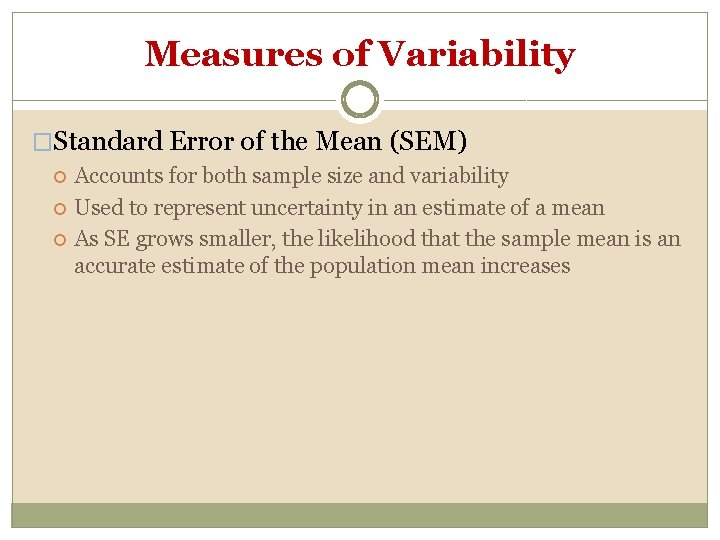Measures of Variability �Standard Error of the Mean (SEM) Accounts for both sample size and variability Used to represent uncertainty in an estimate of a mean As SE grows smaller, the likelihood that the sample mean is an accurate estimate of the population mean increases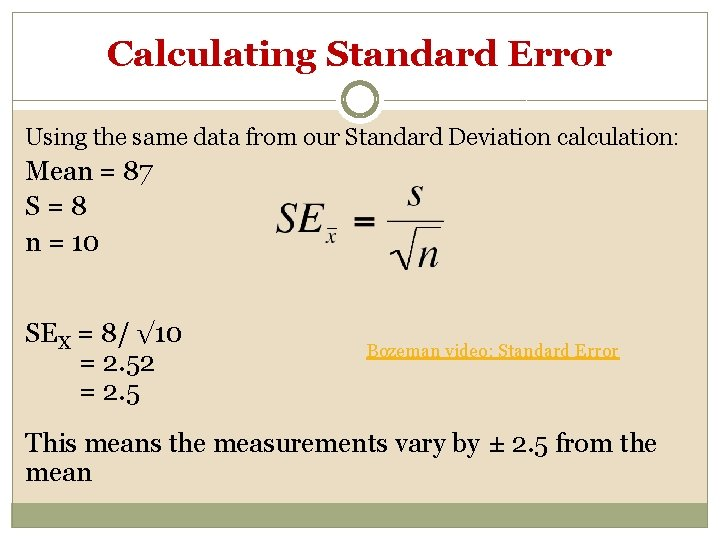Calculating Standard Error Using the same data from our Standard Deviation calculation: Mean = 87 S=8 n = 10 SEX = 8/ √ 10 = 2. 52 = 2. 5 Bozeman video: Standard Error This means the measurements vary by ± 2. 5 from the mean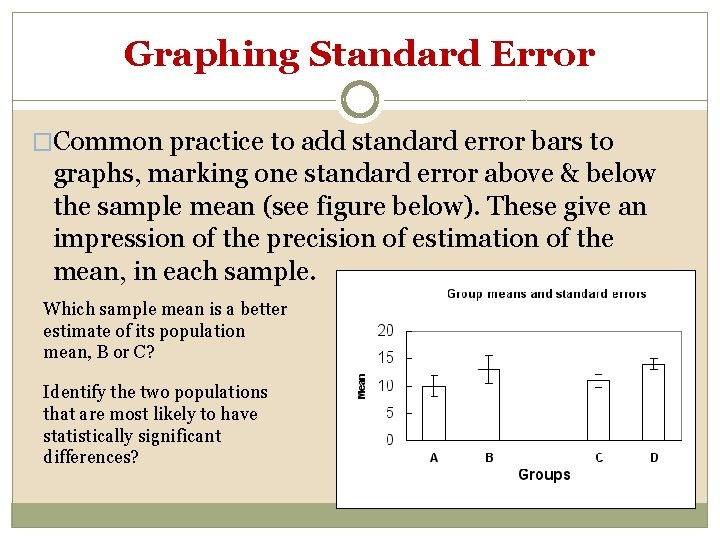Graphing Standard Error �Common practice to add standard error bars to graphs, marking one standard error above & below the sample mean (see figure below). These give an impression of the precision of estimation of the mean, in each sample. Which sample mean is a better estimate of its population mean, B or C? Identify the two populations that are most likely to have statistically significant differences?